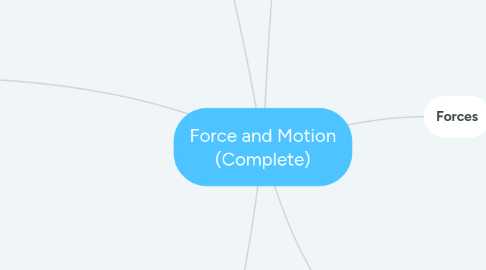# Force and Motion (Complete)Coert Van Zijl
Começar. É Gratuito
ou inscrever-se com seu endereço de e-mail1444
1107
683
150
Force and Motion (Complete)## 1. Motion

### 1.1. Speed

1.1.1. Average speed:

1.1.1.1. Average speed = distance / time

### 1.2. Velocity

1.2.1. Definition:

1.2.1.1. the speed of an object in a particular direction

### 1.3. Acceleration

1.3.1. Definition:

1.3.1.1. the rate at which velocity changes over time

1.3.2. Average acceleration:

1.3.2.1. (final velocity - starting velocity) / time it takes to change velocity

## 2. Forces

### 2.1. What is a force

2.1.1. a push or a pull

### 2.2. SI unit of force (unit of measurement)

2.2.1. Newton (N)

### 2.3. Net force

2.3.1. Forces in the same direction

2.3.1.1. Add forces together to get the net force

2.3.2. Forces in different direction

2.3.2.1. you subtract the smaller force from the bigger force. the net force will be in the direction of the bigger force

### 2.4. Balanced forces

2.4.1. the net force is 0N

### 2.5. Unbalanced forces

2.5.1. the net force is not 0N, and it produces motion.

## 3. Friction

### 3.1. Source of friction

3.1.1. Surface

3.1.1.1. the hills and valleys of the 2 surfaces have hills and valleys. These stick to each other.

3.1.1.2. rougher surfaces have more hills and valleys than smoother surfaces.

3.1.2. Force

3.1.2.1. friction depends on the force pushing surface together

3.1.2.2. a bigger force will cause an increased friction

### 3.2. Types of friction

3.2.1. Kinetic friction

3.2.1.1. Friction between moving objects

3.2.1.2. a force that is applied but does not cause the object to move

3.2.2. Static friction

### 3.3. Reducing friction

3.3.1. 1. Lubricants

3.3.2. 2. Rolling instead of sliding

3.3.3. 3. Smoother surface

### 3.4. Increasing friction

3.4.1. 1. Make surfaces rougher

3.4.2. 2. Increase the force between the surfaces

## 4. Gravity

### 4.2. Law of Universal Gravitation

4.2.1. All objects attract each other through gravitational force. The size of the force depends on the masses and distance apart of the objects.

4.2.2. Gravitational force increase as mass increases

4.2.3. Gravitational force decrease as distance increases

### 4.3. Weight

4.3.1. The measure of gravitational force on an object

4.3.2. Unit of measure: Newton

4.3.3. Measured with a spring scale

### 4.4. Mass

4.4.1. Mass is the amount of matter in an object

4.4.2. Unit of Measure: kilogram (kg)

4.4.3. Measured with balance

## 5. Gravity and Motion

### 5.1. Gravity and Acceleration

5.1.1. All objects are the same. Because...

5.1.2. Acceleration due to gravity

5.1.2.1. all objects accelerate at 9.8 m per second per second (m/s2)

### 5.2. Air resistance

5.2.1. Force of gravity - force of air resistance = net force

5.2.2. Terminal velocity:

5.2.3. Definition:

5.2.4. Free fall

### 5.3. Orbits

5.3.1. Two motions combine to cause orbits

5.3.1.1. Free fall

5.3.1.2. velocity forward

5.3.2. Any object in orbit has an unbalanced force working in on it - centripetal force

### 5.4. Projectile motion

5.4.1. Definition:

5.4.2. 2 components:

5.4.2.1. Horizontal motion

5.4.2.2. vertical motion

## 6. Newton's Laws of Motion

### 6.1. Newton First Law

6.1.1. Definition of law

6.1.2. Inertia

6.1.3. Mass and Inertia

### 6.2. Second Law of Motion

6.2.1. Definition:

6.2.2. Part 1: Acceleration depends on mass

6.2.3. Part 2: Acceleration depends on Force

6.2.4. Expressing law mathematically:

### 6.3. Third law of Motion

6.3.1. Definition: